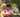### JavaScript Numbers

January 26, 2020

JavaScript Numbers

JavaScript has only one type of number. Numbers can be written with or without decimals. Extra large or extra small numbers can be written with scientific (exponent) notation:

``````var x = 3.14;    // A number with decimals
var y = 3;       // A number without decimals
var x = 123e5;    // 12300000
var y = 123e-5;   // 0.00123``````

### JavaScript Numbers are Always 64-bit Floating Point

Unlike many other programming languages, JavaScript does not define different types of numbers, like integers, short, long, floating-point etc. JavaScript numbers are always stored as double precision floating point numbers, following the international IEEE 754 standard. This format stores numbers in 64 bits, where the number (the fraction) is stored in bits 0 to 51, the exponent in bits 52 to 62, and the sign in bit 63.

Integers (numbers without a period or exponent notation) are accurate up to 15 digits.

``````var x = 999999999999999;   // x will be 999999999999999
var y = 9999999999999999;  // y will be 10000000000000000``````

JavaScript uses the + operator for both addition and concatenation. Numbers are added. Strings are concatenated.

``````var x = 10;
var y = 20;
var z = x + y;           // z will be 30 (a number)

var x = "10";
var y = "20";
var z = x + y;           // z will be 1020 (a string)``````

If you add a number and a string, the result will be a string concatenation:

``````var x = 10;
var y = "20";
var z = x + y;           // z will be 1020 (a string)``````

Examples :

``````var x = 10;
var y = 20;
var z = "The result is: " + x + y;   // The result is: 1020

var x = 10;
var y = 20;
var z = "30";
var result = x + y + z; // 3030``````

JavaScript strings can have numeric content. JavaScript will try to convert strings to numbers in all numeric operations:

``````var x = "100";
var y = "10";
var z = x / y;       // z will be 10

var x = "100";
var y = "10";
var z = x * y;       // z will be 1000

var x = "100";
var y = "10";
var z = x - y;       // z will be 90``````

### NaN - Not a Number

NaN is a JavaScript reserved word indicating that a number is not a legal number.

``````var x = 100 / "Apple";  // x will be NaN (Not a Number)
var x = 100 / "10";     // x will be 10``````

You can use the global JavaScript function isNaN() to find out if a value is a number:

``````var x = 100 / "Apple";
isNaN(x);               // returns true because x is Not a Number``````

Watch out for NaN. If you use NaN in a mathematical operation, the result will also be NaN:

``````var x = NaN;
var y = 5;
var z = x + y;         // z will be NaN``````

Or the result might be a concatenation:

``````var x = NaN;
var y = "5";
var z = x + y;         // z will be NaN5``````

NaN is a number: typeof NaN returns number:

``typeof NaN;            // returns "number"``

### Infinity

Infinity (or -Infinity) is the value JavaScript will return if you calculate a number outside the largest possible number.

``````var myNumber = 2;
while (myNumber != Infinity) {   // Execute until Infinity
myNumber = myNumber * myNumber;
}``````

Division by 0 (zero) also generates Infinity:

``````var x =  2 / 0;       // x will be Infinity
var y = -2 / 0;       // y will be -Infinity``````

JavaScript interprets numeric constants as hexadecimal if they are preceded by 0x.

``var x = 0xFF;        // x will be 255``

By default, JavaScript displays numbers as base 10 decimals. But you can use the toString() method to output numbers from base 2 to base 36.

Hexadecimal is base 16. Decimal is base 10. Octal is base 8. Binary is base 2.

``````var myNumber = 32;
myNumber.toString(10);  // returns 32
myNumber.toString(32);  // returns 10
myNumber.toString(16);  // returns 20
myNumber.toString(8);   // returns 40
myNumber.toString(2);   // returns 100000``````

### Numbers Can be Objects

Normally JavaScript numbers are primitive values created from literals:

``var x = 123;``

But numbers can also be defined as objects with the keyword new:

``var y = new Number(123);``
``````var x = 123;
var y = new Number(123);

// typeof x returns number
// typeof y returns object``````
``````var x = 500;
var y = new Number(500);
// (x == y) is true because x and y have equal values

var x = 500;
var y = new Number(500);
// (x === y) is false because x and y have different types

var x = new Number(500);
var y = new Number(500);
// (x == y) is false because objects cannot be compared``````

Note the difference between (x==y) and (x===y). Comparing two JavaScript objects will always return false.Posted by Tai Lu ( 呂台生 ) who's building useful things.You should follow him on Twitter